Excel 2013 Data Analysis, Part 3: PivotTable

Watch trailer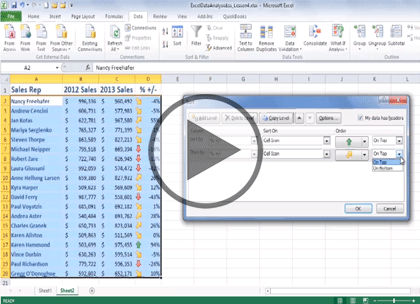Course at a glance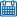Release date 3/17/2015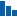Level Intermediate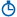Runtime 1h 9m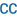Closed captioning N/A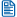Transcript N/A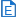eBooks / courseware Included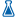Hands-on labs N/A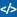Sample code Included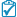Exams N/A

Course description

Master data analysis with Excel 2013 in this three part learning course, Excel 2013 is Microsoft’s industry leading spreadsheet application that allows you to organize, visualize and calculate your data. Lesson coverage includes building formulas and adding functions and conditions to a formula, analyzing financial data to determine loan payments and interest rates, analyzing statistical data and calculating the variance and standard deviation, and sorting and filtering data. You’ll also see an overview of analyzing data with Goal Seek and Scenarios, forecasting data to reveal trends, working with the Analysis ToolPack, and building PivotTables.

Prerequisites

This course assumes some familiarity with Microsoft Excel. This course is part of 3 courses; Excel 2013 Data Analysis: Finance & Stats; Excel 2013 Data Analysis: Sorting and Techniques; and Excel 2013 Data Analysis: Forecast and PivotTable. Please be sure to view the courses in order if you are new to the material.

Meet the expertOur Creative Design courses are presented by experts from Wiley Publishing. Wiley is a global provider of knowledge and knowledge-enabled services that improve outcomes in areas of research, professional practice, and education. They are the publisher of award-winning journals, encyclopedias, books, and online products and services.

Forecast and PivotTable

Trends and Forecasts (21:53)
• Introduction (00:10)
• Plotting a Best-Fit Trendline (03:53)
• The Trend Function (01:45)
• Plotting Forecasted Values (03:21)
• Extending a Linear Trend (02:32)
• Plot an Exponential Trendline (05:13)
• Plot a Logarithmic Trendline (02:22)
• Plotting a Power Trendline (02:23)
• Summary (00:10)
Working with Analysis ToolPak (24:23)
• Introduction (00:10)
• Calculating a Moving Average (02:38)
• Comparing Variances (03:50)
• Calculating Correlation (03:19)
• Running a Regression Analysis (03:47)
• Determining Rank & Percentile (03:04)
• Descriptive Statistics (03:55)
• Summary (00:10)
PivotTables (22:57)
• Introduction (00:10)
• Building a PivotTable (05:25)
• A PivotTable from External Data (03:28)